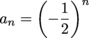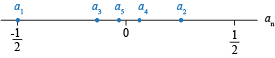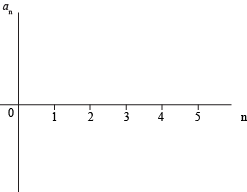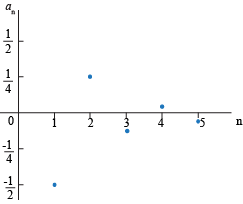Study Guide

# Sequences - Visualizing Sequences

## Visualizing Sequences

For those who like pictures better than formulas, we can visualize sequences on number lines and on graphs. For those who like Kit Kats, we can visualize a giant Kit Kat bar. Either way, creating an image will help us understand better how some sequences behave.

### Numberlines

Some sequences are well-behaved like well-trained dogs, while others are as unpredictable as wild tigers. If we plot the terms of a sequence on a number line, we can get some intuition for what the terms of the sequence are doing.

### Sample Problem

Plot the first five terms of the sequence, starting at n = 1, on a number line.

The first five terms arePlotting these on a number line, we getWe can see that as n gets larger, the terms of the sequence are clumping around 0.

Even if we don't label the terms an on the number line, we can still tell something about what the sequence is doing.

• ### 2-D Graphs

We have all had sliced bread. It's been around since 1928. Two-dimensional graphs have been around for a while, too. While number lines are nice, we can't tell which dots go with which terms. Since we know as much about 2-D graphs as we do sliced bread, we may as well use them and see what happens.

To graph a sequence on a 2-D graph, we put n on the horizontal axis and an on the vertical axis.For each term an of the sequence we graph the point (n, an).

### Sample Problem

Plot the first five terms of the sequence, starting at n = 1, on a graph.